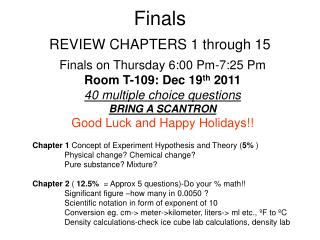DownloadDownload PresentationFinals

# Finals

Download Presentation## Finals

- - - - - - - - - - - - - - - - - - - - - - - - - - - E N D - - - - - - - - - - - - - - - - - - - - - - - - - - -
##### Presentation Transcript

1. 0 Finals REVIEW CHAPTERS 1 through 15 Finals on Thursday 6:00 Pm-7:25 Pm Room T-109: Dec 19th 2011 40 multiple choice questions BRING A SCANTRON Good Luck and Happy Holidays!! Chapter 1 Concept of Experiment Hypothesis and Theory (5% ) Physical change? Chemical change? Pure substance? Mixture? Chapter 2 ( 12.5% = Approx 5 questions)-Do your % math!! Significant figure –how many in 0.0050 ? Scientific notation in form of exponent of 10 Conversion eg. cm-> meter->kilometer, liters-> ml etc., ºF to ºC Density calculations-check ice cube lab calculations, density lab

2. Mass # 35 Cl 17 At. # 0 • 2.6 The Basic Units of Measurement (memorize power of 10 table) • A. English, metric, SI • B. SI Units (Mass – kg; Length – m; Time – sec, volume L) • C. Prefix Multipliers • milli (m) 0.001 • centi (c) 0.01 • kilo (k) 1000 • Mega (M) 1,000,000 • 2.7 Density (D= Mass/Volume) • Chapter 3 (7.5%) • Symbols for elements (from periodic table) • No. protons, no. neutrons, no. electrons, charge problem • Atomic Number = Number of protons • Mass Number = # of Protons + # of neutrons • Isotopes, writing in the 14C convention • Average atomic mass calculation • Chapter 4,9,10 (7.5%) • electronic configuration of atoms and ions • valence electrons • orbital, how many electrons can sub-shells have? S-2, p-6, d? etc.

3. 0 Chapter 5 and 6,11 (25%)- 10 Questions! How many atoms in some chemical formula Al (NO3)3 Ans:13 Correct formulas for chemicals, polyatomic ions- 4 questions Names of compounds molecular and ionic compounds Correct Lewis structure Molecular geometry –important-2 questions! Type of bonding? Ionic, Polar covalent, non polar covalent etc. Polar- non polar??, Chapter 7 (5%) Types of reaction? Displacement, dissociation etc Balancing equation- write the coefficients? Chapter 8,9 –(15%)- 6 questions! How many moles, number of atoms/molecules ? Empirical formula- easy one Molecular formulas- Ratio Calculations in chemical reaction how much reactant will produce how much product, moles to moles, grams to grams etc (3 questions)- STOICHIOMETRY

4. Chapter 13 (5%) • Polar dissolves polar and non polar dissolves non polar • Intermolecular forces are strong will result in high boiling point • H-bonding INTER molecular bond between H and O or F or N • Electrolytes and metallic bonding; London dispersion forces, • dipole-dipole forces • Chapter 12 – Gases –(5%) • Boyle’s law relation of P and V • Charles law relation of V and T • Avogadro's law relation of V and N • combined gas law PV=nRT relationship • P ∞T, P ∞n (pressure is directly proportional to T, and n) • P ∞1/V (pressure is inversely proportional to V) • Chapter 14 (5%) • Mass percentage • Molarity = moles /Litres solution • Molarity and number of moles in solution • Titration: MaVa = MbVb; Dilution • Chapter 15 (10%) • Weak and strong electrolytes (refer to lab): NEED IONS!! • Acids produce H+and Bases produce OH-concepts • pH<7 : Acid; pH>7 bases, pH=7 neutral; Strong acid or strong base • Buffers: Maintain pH 0

5. 0 • 6.023x1023ATOMS = 1 mole OF ATOMS • weighs At. Mass in g • 6.023x1023MOLECULES = 1 mole OF MOLECULES • weighs Molar mass in g • Suppose the molecule has formula A2B • then • 1 mole of A2B consists of 6.023x1023MOLECULES of A2B and • 2 X 6.023x1023ATOMS of A • weighs 2 X At. Mass of A in g • 1 X 6.023x1023ATOMS of B • weighs 1 X At. Mass of B in g

6. 0 Important Formulas to memorize • Chapter 2 – Density, temperature conversion, powers of 10 • Chapter 3,4 – At .#, mass#, protons, neutrons, e-, isotopes, ions • Chapter 9,10 – Valence electrons, electron config. • Chapter 5,6 – Formulas of compounds, Lewis structure rules • Chapter 11 – VSEPR shapes of molecules, polarity, dipole • Chapter 7 – Balancing chemical equations, Single-double displacement reactions • Chapter 8 – Moles? Avogadro number? Molar mass, Stoichiometry Start from balanced equation, moles of A to moles of B? grams of A to grams of B? etc • Chapter 13 –, Concept of inter-molecular bonding, H-bond • Chapter 12 – Gas laws, relation of P vs V, T & n Temp in Kelvin!! • Chapter 14 – Molarity = moles/L of solution, % composition = • Chapter 15 – pH = -log([H+]), [OH-] = Kw/[H+], pH calculations Titrations 1:1 MaVa = MbVb Titrations naMaVa = nbMbVb;